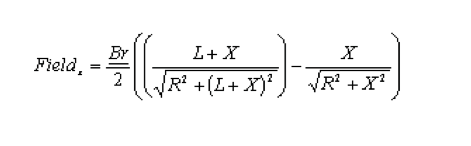WHAT IS THE GOVERNING EQUATION FOR FIELD STRENGTH RELATIVE TO DISTANCE?

# WHAT IS THE GOVERNING EQUATION FOR FIELD STRENGTH RELATIVE TO DISTANCE?

## For a circular magnet with a radiusof R and Length L, the field at the centerline of the magnet a distance X fromthe surface can be calculated by the following formula (where Br is theResidual Induction of the material):